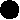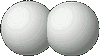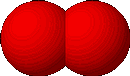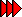# What does this chemical formula mean

### Element symbols - chemical formulas

Chemists use an international sign language. With it chemical reactions can be represented in all languages ​​equally. It includes symbols, formulas and equations.

Element symbols are e.g. S, Fe, O, H, N.

An element symbol has two meanings:

1. Abbreviation for the name of an element
2. Abbreviation for 1 atom of the element

Example:

The element symbol S has two meanings:

1. Abbreviation for sulfur
2. Abbreviation for 1 atom of sulfur

Chemical formulas are e.g. H2O, FeS, CuO, ZnS, HgO.

A chemical formula has three meanings:

1. Abbreviation for the name of a chemical compound
2. stands for 1 molecule of the chemical compound
3. indicates the atomic number ratio of the atoms contained in the molecule

Example:

The chemical formula H2O has three meanings:

1. Abbreviation for water = hydrogen oxide
2. Abbreviation for 1 molecule of water
3. One molecule of water contains the elements hydrogen and oxygen in a ratio of 2: 1.

A large number in front of a chemical formula indicates the number of molecules.

Examples:

2 feet means 2 atoms of iron

Fe Fe5 CuO means 5 molecules of copper oxide

A small number Behind an element symbol gives the Number of atoms at that in a molecule connected with each other are.

ExamplesH2means a hydrogen molecule made up of two hydrogen atoms linked together.O2means an oxygen molecule made up of two oxygen atoms linked together.

3 H.2 means three hydrogen molecules made up of two eachinterconnected hydrogen atoms exist.

further